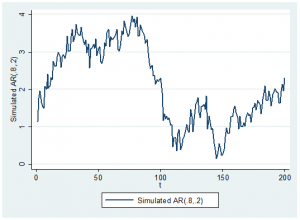22
Jun 17

## Autoregressive–moving-average (ARMA) modelsAutoregressive–moving-average (ARMA) models were suggested in 1951 by Peter Whittle in his PhD thesis. Do you think he played with data and then came up with his model? No, he was guided by theory. The same model may describe visually very different data sets, and visualization rarely leads to model formulation.

Recall that the main idea behind autoregressive processes is to regress the variable on its own past values. In case of moving averages, we form linear combinations of elements of white noise. Combining the two ideas, we obtain the definition of the autoregressive–moving-average process:

(1)$y_t=\mu+\beta_1y_{t-1}+...+\beta_py_{t-p}+ \theta_1u_{t-1}+...+\theta_qu_{t-q}+u_t$$=\mu+\sum_{i=1}^p\beta_iy_{t-i}+\sum_{i=1}^q\theta_iu_{t-i}+u_t.$

It is denoted ARMA(p,q), where p is the number of included past values and q is the number of included past errors (AKA shocks to the system). We should expect a couple of facts to hold for this process.

If its characteristic polynomial$p(x)=1-\beta_1x-...-\beta_px^p$ has roots outside the unit circle, the process is stable and should be stationary.

A stable process can be represented as an infinite moving average. Such a representation is in fact used to analyze its properties.

The coefficients of the moving average part (the thetas) and the constant$\mu$ have no effect on stationarity.

The quantity$\partial y_t/\partial y_{t-1}=\beta_1$ can be called an instantaneous effect of$y_{t-1}$ on$y_t$. This effect accumulates over time (the value at$t$ is influenced by the value at$t-1$, which, in turn, is influenced by the value at$t-2$ and so on). Therefore the long-run interpretation of the coefficients is complicated. Comparison of Figures 1 and 2 illustrates this point.

Exercise. For the model$y_t=\mu+\beta_1y_{t-1}+u_t$ find the mean$Ey_t$ (just modify this argument).Figure 1. Simulated AR processFigure 2. Simulated MA

Question. Why in (1) the current error$u_t$ has coefficient 1? Choose the answer you like:

1) We never used the current error with a nontrivial coefficient.

2) It is logical to assume that past shocks may have an aftereffect (measured by thetas) on the current$y_t$ different from 1 but the effect of the current shock should be 1.

3) Mathematically, the case when instead of$u_t$ we have$\theta_0u_t$ with some nonzero$\theta_0$ can be reduced to the case when the current error has coefficient 1. Just introduce a new white noise$v_t=\theta_0u_t$ and rewrite the model using it.